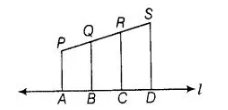# In figure, PA, QB, RC and SD are

Question:

In figure, PA, QB, RC and SD are all perpendiculars to a line i, AB = 6 cm, BC = 9 cm, CD = 12 cm and SP = 36 cm. Find PQ, QR and RS.Solution:

Given, AS = 6 cm, BC = 9 cm, CD = 12 cm and SP = 36 cm

Also, PA, QB, RC and SD are all perpendiculars to line l.

PA || QS|| SC || SD

By basic proportionality theorem,

$P Q: Q R: R S=A B: B C: C D$

$=6: 9: 12$

Let $\quad P Q=6 x, Q R=9 x$ and $R S=12 x$

Since, length of $P S=36 \mathrm{~km}$

$\therefore$ $P Q+Q R+R S=36$

$\Rightarrow \quad 6 x+9 x+12 x=36$

$\Rightarrow \quad 27 x=36$

$\therefore \quad x=\frac{36}{27}=\frac{4}{3}$

$Q R=9 x=9 \times \frac{4}{3}=12 \mathrm{~cm}$

and $R S=12 x=12 \times \frac{4}{3}=16 \mathrm{~cm}$